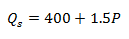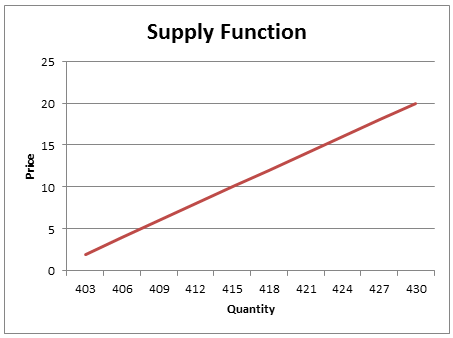# Supply Function and Supply Curve

Supply is the ability and willingness of the firms to sell a specific quantity of a good or service at a given price in a given time period. This will depend on many factors such as the cost of machinery, labor cost, price of the product, prices of related products, number of firms producing the product, etc.

### Supply Function

When the quantity supplied is expressed only as a function of the price of the product, other factors remaining constant, it is called a supply function. The following is a simple supply function:The positive sign indicates that the price and quantity supplied are positively related, i.e., when the price increases, the quantity supplied will also increase. In the above example, for every \$1 increase in price, the quantity supplied will increase by 1.5 units.

### Supply Curve

The information from the supply function can be plotted as a simple graph with quantity supplied on x-axis and price on y-axis. This is called a supply curve.

The equation plotted is the inverse supply function, P = f(Qs)

A point on a direct supply curve can be interpreted as follows:

• Maximum amount of a good that will be offered for sale at a given price
• Minimum price necessary to induce producers to voluntarily offer a particular quantity for saleThe supply curve illustrates the law of supply. The law of supply states that when the price of a good rises, and everything else remains the same, the quantity of the goods supplied will increase.